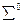Chapter 33, Problem 18PE

Chapter
Section
Textbook Problem

The principal decay mode at the sigma zero is(a) What energy is released?(b) Considering the quark structure of the two baryons, does it appear that theis an excited state of the(c) Verify that strangeness, charge, and baryon number are conserved in the decay.(d) Considering the preceding and the short lifetime, can the weak force be responsible? State why or why not.

To determine

(b)

Whether Σ0 is an excited state of Λ0.

Explanation

Given:

The decay mode of Σ0 is given by, Σ0Λ0+γ

Calculation:

Quark structure of Σ0

=uds

Quark structure of Λ0

=uds

The decay mode of Σ0 i

To determine

(c)

The conservation of charge baryon number and strangeness for the decay Σ0Λ0+γ.

To determine

(d)

Whether weak force be responsible for the decay of Σ0Λ0+γ.

Still sussing out bartleby?

Check out a sample textbook solution.

See a sample solution

The Solution to Your Study Problems

Bartleby provides explanations to thousands of textbook problems written by our experts, many with advanced degrees!

Get Started